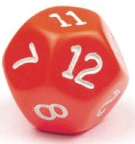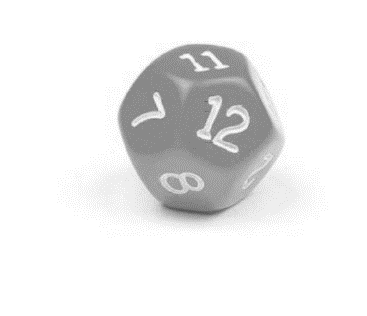Chapter 9.1, Problem 1CP### Calculus: An Applied Approach (Min...

10th Edition
Ron Larson
ISBN: 9781305860919

#### Solutions

Chapter
Section### Calculus: An Applied Approach (Min...

10th Edition
Ron Larson
ISBN: 9781305860919
Textbook Problem
1 views

# Checkpoint 1 Worked-out solution available at LarsonAppliedCalculus.comAn experiment consists of rolling a twelve-sided die, similar to the one shown at the left.a. What is the sample space?b. Describe the event corresponding to rolling a number greater than 7.(a)

To determine

The sample space of an experiment consists of rolling a twelve-sided die, similar to the one shown at given figureExplanation

Given Information:

An experiment consists of rolling a twelve-sided die, similar to the one shown at given figure

The sample space is the total possible outcome of an experiment.

So, for an experiment consists of rolling a twelve-sided die, the sample space S consist of twelve outcome, which can be represented by numbers 1 to 12.

S={1,2,3,

(b)

To determine

The event corresponding to rolling a number greater than 7 for an experiment consists of rolling a twelve-sided die, similar to the one shown at given figure### Still sussing out bartleby?

Check out a sample textbook solution.

See a sample solution

#### The Solution to Your Study Problems

Bartleby provides explanations to thousands of textbook problems written by our experts, many with advanced degrees!

Get Started

#### Evaluate the expression sin Exercises 116. (2)3

Finite Mathematics and Applied Calculus (MindTap Course List)

#### In Problems 7-34, perform the indicated operations and simplify. 11.

Mathematical Applications for the Management, Life, and Social Sciences

#### True or False: is a rational function.

Study Guide for Stewart's Single Variable Calculus: Early Transcendentals, 8th

#### The equation of the tangent plane to for is:

Study Guide for Stewart's Multivariable Calculus, 8th

#### Self Check Evaluate: k=15(k21).

College Algebra (MindTap Course List)

#### Why is it necessary to have a stable pattern within a phase?

Research Methods for the Behavioral Sciences (MindTap Course List)